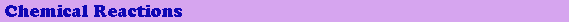### In the state the spheres (ions) are arranged so that any particular ion is surrounded by the oppositely charged ion. What ever pattern which arises depends on several factors which we will not be discussing at this point in our class. Below is a picture of a model of a simple ionic compound. Notice the spheres (ions) of different sizes and how each sphere (ion) is surrounded by the other spheres (ion).### Consider the balanced equation### To answer this question we need to convert grams to mol. Since 1 mol of has a mass of 180 g we can set up the conversion as;### The molar mass of N2O5 is 108 g­mol-1. The sample is 45.6 g so we know there is less than a mol since a mol of N2O5 weighs 108 g. To calculate the number of moles we setup the problem in the following way;### Now we can determine the number of nitrogen atoms;